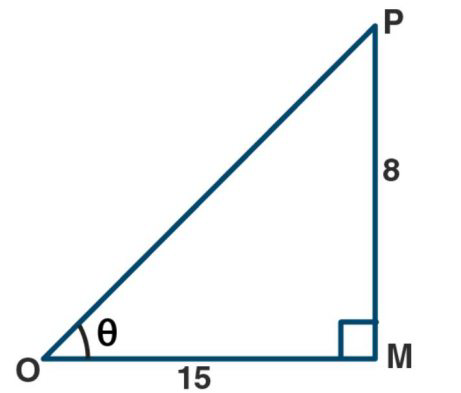# ML Aggarwal Solutions Class 9 Mathematics Solutions for Trigonometric Ratios Exercise 17 in Chapter 17 - Trigonometric Ratios

If O is an acute angle and tan = 8/15 then find sec + cosec

Given tan θ = 8/15

θ is an acute angle

in the figure triangle, OMP is a right-angled triangle,

\angle \mathrm{M}=90^{\circ} \text { and } \angle \mathrm{Q}=\thetaTan θ = PM/OL = 8/15

Therefore, PM = 8, OM = 15

\begin{aligned} &\text { But } \mathrm{OP}^{2}=\mathrm{OM}^{2}+\mathrm{PM}^{2} \text { using Pythagoras theorem, }\\ &=15^{2}+8^{2}\\ &=225+64\\ &=289\\ &=17^{2} \end{aligned}

Therefore, OP = 17

Sec θ = OP/OM

= 17/15

Cosec θ = OP/PM

= 17/8

Now,

Sec θ + cosec θ = (17/15) + (17/8)

= (136 + 255)/ 120

= 391/120

Related Questions
Exercises

Lido

Courses

Teachers

Book a Demo with us

Syllabus

Maths
CBSE
Maths
ICSE
Science
CBSE

Science
ICSE
English
CBSE
English
ICSE
Coding

Terms & Policies

Selina Question Bank

Maths
Physics
Biology

Allied Question Bank

Chemistry
Connect with us on social media!